Multiple depots vehicle routing based on the ant colony with the genetic algorithmMultiple depots vehicle routing based on the ant colony with the genetic algorithm

ChunYing Liu, Jijiang Yu*

Heze university (China)

Accepted: May 2013

liu, C., & Yu, J. (2013). Multiple depots vehicle routing based on the ant colony with the genetic algorithm. Journal of Industrial Engineering and Management, 6(4), 1013-1026. http://dx.doi.org/10.3926/jiem.747

---------------------

Abstract:

Purpose: the distribution routing plans of multi-depots vehicle scheduling problem will increase exponentially along with the adding of customers. So, it becomes an important studying trend to solve the vehicle scheduling problem with heuristic algorithm. On the basis of building the model of multi-depots vehicle scheduling problem, in order to improve the efficiency of the multiple depots vehicle routing, the paper puts forward a fusion algorithm on multiple depots vehicle routing based on the ant colony algorithm with genetic algorithm.

Design/methodology/approach: to achieve this objective, the genetic algorithm optimizes the parameters of the ant colony algorithm. The fusion algorithm on multiple depots vehicle based on the ant colony algorithm with genetic algorithm is proposed.

Findings: simulation experiment indicates that the result of the fusion algorithm is more excellent than the other algorithm, and the improved algorithm has better convergence effective and global ability.

Research limitations/implications: in this research, there are some assumption that might affect the accuracy of the model such as the pheromone volatile factor, heuristic factor in each period, and the selected multiple depots. These assumptions can be relaxed in future work.

Originality/value: In this research, a new method for the multiple depots vehicle routing is proposed. The fusion algorithm eliminate the influence of the selected parameter by optimizing the heuristic factor, evaporation factor, initial pheromone distribute, and have the strong global searching ability. The Ant Colony algorithm imports cross operator and mutation operator for operating the first best solution and the second best solution in every iteration, and reserves the best solution. The cross and mutation operator extend the solution space and improve the convergence effective and the global ability. This research shows that considering both the ant colony and genetic algorithm together can improve the efficiency multiple depots vehicle routing.

Keywords: genetic algorithm, ant colony algorithm, multiple depots, vehicle routing, fusion algorithm

---------------------

1. Introduction

In recent years, logistics distribution is getting increasingly important in playing role of constructing country economy. How to increase distribution efficiently and reduce its cost is becoming a hot research topic for many corporations and researchers of science. Multi‑Distribution Center Vehicle Routing Problem becomes intelligent logistics scheduling of a research hot spots (Crevier, Cordeau & Laporte, 2007). There are two methods for solving the multi-depot vehicle routing problem. One is the exact algorithm, and the other is the heuristic algorithm. The exact algorithm includes the branch and bound method, the cutting plane method, the network flow algorithm, the dynamic programming, etc. The heuristic algorithm is structured by the experience, so its solution is satisfied and isn’t necessarily the optimal under the acceptable time and space for the multiple depots vehicle routing (Nagy & Salhi, 2005). The heuristic algorithm of vehicle routing has become an important direction, and a large number of algorithms have emerged. For example, Clark and Wright propose the saving method, the scanning method of Gillett and Miller. Bramelt and Simchi-Levi propose LBH method based on conversion of the location problem. The other methods include the genetic algorithm, the taboo search, the simulated annealing algorithm, intelligent heuristic algorithm, etc (Chan, Carter & Burnes, 2001).

In recent years, the application of ant colony optimization algorithm (ACO) to solve the vehicle routing problem has become a research focus. Zhong-Yun Liu improves the basic ant colony algorithm, and adjusts dynamically volatile factor for solving a dynamic vehicle routing problem with time Windows. Rui-Chen Yang, Yong-Fu Zhou improve the ant colony algorithm from visibility, the information concentration and the parameters, and adopt the exchange method to search for solving the logistics distribution vehicle routing problem. The Ant Colony Algorithm is a population-based heuristic algorithm to solve complicated combinatorial optimization problems (Gulczynski, Golden & Wasil, 2011). As a swarm intelligent technology, ACO has strong ability of the global optimization and the parallelism, and it can get results quickly and have obvious advantages in the dynamic optimization. However, the ant colony algorithm has weakness in the convergence effective. Using the nature rule of survival of the fittest, the genetic algorithm can count the initial solution in the beginning and get the better feasible solution in the process, and gets rid of the non-feasible solution. Genetic Algorithm has strong ability for solving complicated nonlinear problem, and has been successfully applied in pattern recognition and intelligent control, etc. In order to improve the convergence effective and the global ability of basic ant colony algorithm, a hybrid algorithm is proposed in the paper, which is a combination of genetic algorithm and ant colony algorithm. The genetic algorithm optimizes the parameters of the ant colony algorithm by cross operator and mutation operator. The fusion algorithm reserves the best solution in every evolution. The manipulation of reserving the intersection accelerates convergence speed. The manipulation of importing cross operator and mutation operator broads the solution space and improves the global ability of the algorithm.

2. Mathematical model of the multi-logistics center distribution

In the study on the multi-logistics center distribution, the network nodes have the parking lot, distribution center and the users. The cost between the station yard, the distribution sites, and the users is the weight any two vertices.

H, M—Are respectively the number of the distribution center and the distribution sites

qi—Demand of customer i (Kuo & Wang, 2012).

[ai,bi]—Time range that the customers demand

dij—Distance between point i and j

tij—Time between point i and j

si—Time the vehicle arrives at depot i

tiw— Waiting time at depot i

Qk—Maximum freight volume of vehicle k

Dk—Maximum distance of vehicle k

nk—Consumer number of vehicle k

Rk = {rk1, rk2,...rkn}—is the path set, and the rki is the consumer sequence.

The mathematical model of the multi-logistics center distribution is as follows (Lin & Kwok, 2006).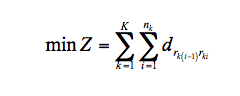(1)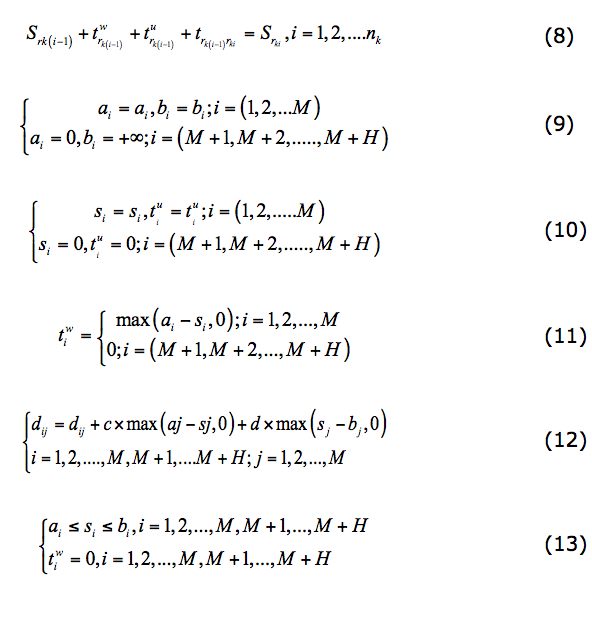Constraints (2) guarantee the starting and ending points is the same, while constraints (3) and (4) state that all paths is parallel and its number doesn’t exceed the clients. Constraints (5) give that the multi-logistics centers haven’t assignment. Constraints (6) guarantee the demand amount doesn’t exceed the load. Constraints (7) and (8) are the route length and customers number constraints. Constraints (9) are the limited time. Constraints (10), (11) and (12) are the soft time windows, and Constraints (13) is the hard time windows. The c and d is the penalty coefficient.

3. Ant Colony algorithms and the genetic algorithm

3.1. Ant colony algorithm

Ant Colony Algorithm (ACA) algorithm is a meta-heuristic algorithm for the approximate solution of combinatorial optimization problems that has been inspired by the foraging behavior of real ant colonies. In ACA algorithm, the computational resources are allocated to a set of relatively simple agents that exploit a form of indirect communication mediated by the environment to construct solutions to the finding the shortest trajectory from ant nest to a considered problem. The probability that the foraging ants move from point i to j is as follows.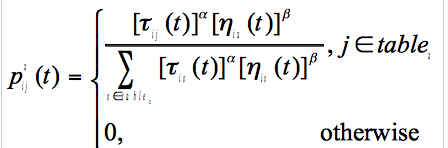(14)

The formula (14) combines the diversity and the feedback of the foraging ants, and τij(t), ηij(t) is respectively the pheromone function and heuristic function. α, β are the heuristic factor, and the diversity and feedback are shown by the different α, β. The pheromone updating rules are as follows when the ants forage at t and t+n time.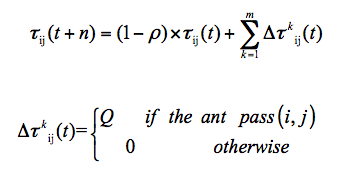(15)

ρ is the volatile coefficient, and 1-ρ is the residual coefficient. Δτkij(t) is the increasing amount of the pheromone between the I and the J.

3.2. The genetic algorithm

The genetic algorithm (GA) was first promulgated by Holland (1975), and it was inspired by the evolution theory. GA is an optimization method with the advantages by the high degree of parallel, random and self-adapting, which based on the survival of the fittest. It represents the problem solving as the evolution of chromosomes. The optimization result is searched by selection, cross and mutation and other genetic operations over the generations. GA is commonly used optimization algorithm, and its encoding technique and genetic operations prove reasonably easy. GA’s optimal result is independent of the restrictive conditions. The implicit parallelism and global searching are two characteristics of GA. Recently, with the development of computer technique, GA has received more and more attention, and it has been used extensively in many fields such as adaptive control, combinatorial optimization, pattern recognition, machine learning, artificial life, management decisions, neural network and VLSI design, etc.

Using the proceeding notions, we now describe the seven steps in the Standard Genetic Algorithm.

Step 1: Select the population with random individuals.

Step 2: Calculate the fitness of each individual.

Step 3: Choose two individuals, and remove the parents from the population.

Step 4: Use a random process to determine whether to perform crossover. If so, refer to the output of the crossover as the children. If not, simply refer to the parents as the children.

Step 5: Mutate the children with probability pm for each bit.

Step 6: Put the two children into an empty set called the new generation.

Step 7: Return to Step 2 until the new generation contains n individuals. Delete one child at random if n is odd. Then replace the old population with the new generation. Return to Step 1.

4. Encoding methods and operation of the chromosome

4.1. Encoding methods

There are the two encoding methods based on the vehicle routing sequence and customer in multiple depots vehicle routing. In this paper, the two encoding methods is comprehensive and modified, and the chromosome is (G1, G2,..., GL), an Gi d includes four parts, start_depot_num, vehicle_num, order_num, end_depot. start_depot_num is the initial station numbers, and vehicle_num is the vehicle numbers. order_num is the serial number, and end_depot is the final station numbers. Gi shows the customers’ vehicles start from start_depot_num to end_depot. order_num is the order of vehicle transportation path.

For example, the structure Chromosome S is S(A13BB13AA11BA12BB12AB11A). The vehicle No. 1 from A travels by A-3-4-1-B and returns to B, and the customers 3, 4, 1 are visited. The vehicle No. 1 from B station travels B-6-5-2-A and returns to A, and the customer 6, 5, 2 are visited. The distribution vehicle uses two cars, and the chromosome’s encoding indicates appropriately the open vehicle routing scheduling problem.

4.2. Population initialization rule

Because the model on the multiple depots vehicle routing contains the constraint conditions, it isn’t feasible for initializing chromosomes by a randomized method. For example, the vehicles must be the same from a starting station to a returning yard, and every car is corresponding to a starting and termination station, etc. Set especially the following rules for the chromosome initialization.

Rule 1: The starting start_depot_num can be all the station numbers, but its number is the same with end_depot, and ensure each station vehicle number is unchanged.

Rule 2: Each vehicle can only correspond to the initial and termination station, and if the first and second of every four gene is certain, and the fourth is also determined.

Rule 3: the first and the fourth is random for every four genes series, and the second belong to Kn (n=1,2,...,N). In addition, the first and fourth value should also follow the rule 1 and 2.

4.3. The crossover operation

The chromosome is (C1, C2,...,CL).

C1 = (A,...,cm,...,cn,...,cx,...,cy,B) and C2 = (A,...,cx,...,cy,...,cm,...,cn,B) represents respectively the first best solution and the second best solution in every iteration. (cm,...,cn), (cx,...,cy) are the cross subset of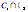. NewC1 and NewC2 are the result of the crossover operation. NewC1 includes C1 and the front of the cross subset in C2, and NewC2 include C2and the front of the cross subset in C1. For example, C1 = (A, 12, 10, 9, 1, 2, 3, 11, 8, 4, 5, 6, 7, B), C2 = (A, 8, 11, 4, 1, 2, 3, 10, 5, 6, 7, B), NewC1 = (A, 8, 4, 1, 1, 12, 10, 9, 1, 2, 3, 5, 6, 7, B), NewC2 = (A, 12, 10, 9, 8, 11, 4, 1, 2, 3, 5, 6, 7, B).

4.4. Mutation operation

The mutation operation can expand the search space, and avoid the local optimal solution. Mutation operator mutates the new individual according to the certain probability. The large probability leads the blindness of the search space, and the small hides the formation of the new genes .This paper takes 0.01 as the mutation rate. The mutation operation adopts the reverse method. C1 = (A, 12, 10, 9, 1, 2, 3, 11, 8, 4, 5, 6, 7, B), and the breaking point is set at 10 and 9. The reverse sequence is inserted, and the result of the mutation operation C1 is (A, 12, 9,10, 1, 2, 3, 11, 8, 4, 5, 6, 7, B).

5. Fusion algorithm of the multiple depots vehicle routing based on the Ant Colony with the genetic algorithm

5.1. Fusion algorithm of the ant colony algorithm with the genetic algorithm

5.1.1 Influence of the parameters in the ant colony algorithm

The performance in Ant colony algorithm depends greatly on the number of ants, pheromone volatile factor, heuristic factor, expect heuristic factor, pheromone strength and so on. Therefore, how to choose effective related parameters is the key of ant colony algorithm.

Influence of the pheromone volatile factors

The volatile factors ρ relates directly to the global search ability and convergence speed in the ant colony algorithm. The parameter ρ reflects the interaction of the individual ants. When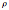is large, the residual pheromone is dominant, and the positive feedback effect is relatively weak. At the same time, the algorithm strengthens randomness search, and the convergence speed is slow. When ρ is small, the positive feedback is relatively strong, and the algorithm abates the randomness. At the same time, the convergence speed fast relatively, but it is easy to fall into the local optimal solution.

Influence of the heuristic factors

The heuristic factor α reflects how important the accumulation of the pheromone is in ant colony algorithm. When α is large, the ants choice the former road by more probability, and the search randomness weaken. The convergence speed slows down when α is small.

Influence of expect heuristic factors

The expectation heuristic factor β reflects the relative important degree of the heuristic information in the ant colony search. β is the apriority knowledge and certainty factor in the optimization. When β is large, the ants choose the shortest path by the more probability. At the same time, the convergence speed accelerates, and the randomness abates. It is easy to fall into local optimal solution. When β is small, the randomness increases, and the convergence speed slows down.

Influence of the pheromone strength

Pheromone strength Q is the total amount of the released pheromone when the ants cycle. When Q is large, the ants has the faster accumulation of the pheromone, so as to strengthen the positive feedback effect. At the same time, the algorithm convergence speeds, and the randomness abates, so it is easy to fall into the local optimum. When Q is small, the randomness increases, but the convergence speed is slows down.

5.1.2. Optimization algorithm of the ant colony algorithm with the genetic algorithm

Due to the uncertainty and the mutual influence of the parameter in the ant colony algorithm, it is difficult how to select the optimal combination of the parameters for solving the problem. There isn’t the perfect theoretical basis up to now, and the result is achieved by many repeated experiment in most cases. The steps that the genetic algorithm optimizes the parameters of the ant colony algorithm are as follows.

Step 1: The chromosome coding of the parameter.

The research indicates that the best range of the parameters is 0.1 ρ 0.99, 0 α 5, 0.1 β 5, 10 ρ 10000. Real number coding has high accuracy, and it is easy to search the large space. This coding operation is simple, and it is suitable for the optimization problem. The chromosome (ρ, α, β, Q) is a parameter combination in the ant colony algorithm.

Step 2: Initialize the population.

According to the range of each parameter, initialize randomly generated population

Step 3: Fitness function.

In order to consider fully the characteristics of the ant colony algorithm, the fitness function is built from the objective function of the solving problem, the ant colony algorithm exploration and development ability.(16)

f(x) is the total target function of the distribution center. Er is the global search ability of the ant colony. fs(x) is the optimum function for all the iterative process, and Ei is the global search ability of the ant colony. fi(x) is current iterative optimal function, and r, rand( ) is the random [0,1].

Step 4: The crossover.

c1 = (ρ, α, β, Q), c2 = (ρ, α, β, Q), according to the following formula for the crossover.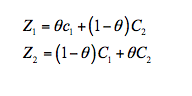(17)

θ is the random [0,1].

Step 5: The mutation.

c = (ρ, α, β, Q) is the real number coding, and choice position k(1 ≤ k ≤ 4) for the mutation. Gene xk is as follows after mutation.

 Sk' = xk +d (18)

δ is the step of the mutation, and it is uniform distribution.

Step 6: Selection strategy.

Fi is the selected probability for individual, and it is the proportion of the fitness value for the individual and the total. The population scale is N, and the selected probability for individual is as follows.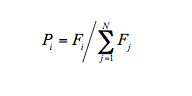(19)

Get the probability, and adopt the rotary wheel method for selection operation.

Step 7: Turn to step 3 if the iterations is the maximum. Otherwise, the algorithm is over.

5.2. Fusion algorithm on multiple depots vehicle routing based on the Ant Colony with the genetic algorithm

The whole algorithm is divided into two stages. The first stage introduces the genetic algorithm with the advantage of the convergence speed and global property, and use it adjust the initial parameters. The second stage executes the ant colony algorithm with the initialized parameters in the first stage, and adds the crossover and the mutation operation for solution of the multiple depots vehicle routing. The fusion algorithm is as follows.

Step 1: Execute the fusion algorithm of the ant colony with the genetic algorithm for setting the initial (ρ, α, β, Q).

Step 2: Initialize H, M, qi (i = 1, 2,..., M+H), Qk (k = 1, 2,..., K), Dk (k = 1, 2,..., K), dij (i,j = 1, 2,..., M, M+1, M+2, …, M+H), [ai, bi], F = (M+H)/2, ρ(t0) = 0.5, ρmax = 0.9, ε = 1.1, h = 0, τij (0) = τmax = (1/(1–ρ)×(1/Fbest)), τmin = τmax / (2(M+H)), NC = 0. Punishment coefficient c, d, NCmax is the maximum of the cycle.

Step 3: Place the n ants at vertexes. If the vertex is the distribution center, set it as the permanent, and add the other distribution center into taboo list. If the vertex isn’t the distribution center, add it into taboo list. route_length is the length of the current path, and the array visited[N] deposits the visited node, and the ants serial numbers k is zero.

Step 4: If k > m, turn to step 8.

Step 5: all nodes i (i = 1, 2, M+H), viisted[i] = 0.

Step 6: Select randomly a depot, start_depot_num is the current serial number, i = start_depot_num.

Step 7: Select the next node j according to the formula (14).

Step 8: If the node j isn’t found, execute all following operation. j= –1. Join the edge (i, start_depot_num) into Route. If all the nodes have visited, the ant completes search. Update the pheromone in local area. k = k + 1, if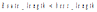, Best_route is Route, and best_length is less than route_length, turn to step 2. If all nodes are visited, qual = 0, turn to step 6.

Step 9: Join edge (i, j) into Route, qual = qual + qi, visited[j] = 1, i = j, turn to step (3);

Step 10: Update the global pheromone according to the formula (15). Calculate the first best path and the second best path. If the iteration is maximum, turn to step 13.

Step 11: The crossover operation for the first best path and the second best path , and update the best path.

Step 12: The mutation operation for the first best path and the second best path, and update the best path. Turn to step (4).

Step 13: End the algorithm.

6. Simulation experiments

Use VC+ + 6.0 to design the program, and select the standard testing Cordeau p01 and p02. Each dataset has four distribution centers, and the customers’ number is 50. There are four cars that the maximum capacity is 80 kg at the P01. The P02 has 2 cars, and the maximum load is 160 kg. There are unlimited in the maximum distance.

In Cordeau standard example, this paper proposes the fusion algorithm of the ant colony with the genetic algorithm (GAACFA) and TS, VNS, CNVNS solves the path length on multiple depots vehicle routing in Table 1 and Table 2.

In order to verify the influence of the genetic algorithm with the ant colony in GAACA, and removes the genetic algorithm to get the ant colony algorithm on multiple depots vehicle routing (ACA). The algorithms set the same parameters and adopt the consistent data, and the results are Table 3 and Table 4.

 Algorithm Best solution (km) Worst solution (km) Average solution (km) TS 592.56 618.43 602.37 VNS 587.63 603.51 596.86 CAVNS 576.87 598.96 590.23 GAACFA 575.43 591.23 582.45

Table 1. GAACFA and the Other Algorithms in Solving Path Length in P01

 Algorithm Best solution (km) Worst solution (km) Average solution (km) TS 481.56 508.36 498.63 VNS 482.56 498.54 492.54 CAVNS 473.53 490.97 485.57 GAACFA 470.42 487.36 476.54

Table 2. GAACFA and the other algorithms in solving path length in P02

 Algorithm Global optimal Local optimal Average convergence algebra Average solution (km) ACA 34 28 78 632.32 GAA 38 26 76 612.34 GAACFA 52 11 72 582.45

Table 3. Performance of the algorithms in P01

 Algorithm Global optimal Local optimal Average convergence algebra Average solution (km) ACA 26 29 96 503.66 GAA 41 24 82 493.64 GAACFA 54 12 71 476.54 ACA 26 29 96 503.66

Table 4. Performance of the algorithms in P02

The global optimal convergence of the GAACFA is more than ACA from the table 3 and 4, and the P01 is 52 times, the P02 is 54 times. The average convergence algebra reflects the convergence speed, and the GAACFA has the more convergence speed and the less average route for adding the genetic algorithm.

7. Conclusions

In this paper, a new approach for improving the efficiency of the multiple depots vehicle routing is proposed. This paper gives the multi-depots vehicle scheduling model and the coding method of the ants. The fusion algorithm eliminate the influence of the selected parameter by optimizing the heuristic factor, evaporation factor, initial pheromone distribute, and have the strong global searching ability. The Ant Colony algorithm imports cross operator and mutation operator for operating the first best solution and the second best solution in every iteration, and reserve the best solution. The cross and mutation operator extend the solution space and improve the convergence effective and the global ability. The results show that the fusion algorithm is more excellent than the other algorithm, and the improved algorithm has better convergence effective and global ability.

In conclusion, this research is believed to improve the efficiency multiple depots vehicle routing. It has been shown that considering the ant colony and genetic algorithm together can improve the working conditions and reduce the efficiency of the multiple depots vehicle routing.

Acknowledgements

This work was financially supported by the science and technology projects of the Shandong province universities (J12LN55).

References

Chan, Y., Carter, W.B., & Burnes, M.D. (2001). A multiple-depot, multiple-vehicle, location-routing problem with stochastically processed demands. Computers & Operations Research, 28(8), 803-826. http://dx.doi.org/10.1016/S0305-0548(00)00009-5

Crevier, B., Cordeau, J.F., & Laporte, G. (2007). The multi-depot vehicle routing problem with inter-depot routes. European Journal of Operational Research, 176(2), 756-773. http://dx.doi.org/10.1016/j.ejor.2005.08.015

Gulczynski, D., Golden, B., & Wasil, E. (2011). The multi-depot split delivery vehicle routing problem: An integer programming-based heuristic, new test problems, and computational results. Computers & Industrial Engineering, 61(3), 794-804. http://dx.doi.org/10.1016/j.cie.2011.05.012

Ho, W., Ho, G.T.S., Ji, P., & Lau, H.C.W. (2007). A hybrid genetic algorithm for the multi-depot vehicle routing problem. Engineering Applications of Artificial Intelligence, 21(4), 548‑557. http://dx.doi.org/10.1016/j.engappai.2007.06.001

Kuo, Y., & Wang, C.C. (2012). A variable neighborhood search for the multidepot vehicle routing problem with loading cost. Expert Systems with Applications, 39, 6949-6954. http://dx.doi.org/10.1016/j.eswa.2012.01.024

Lin, C.K.Y., & Kwok, R.C.W. (2006). Multi-objective metaheuristics for a location-routing problem with multiple use of vehicles on real data and simulated data. European Journal of Operational Research, 175(3), 1833-1849. http://dx.doi.org/10.1016/j.ejor.2004.10.032

Nagy, G., & Salhi, S. (2005). Heuristic algorithms for single and multiple depot vehicle routing problems with pickups and deliveries. European Journal of Operational Research, 162, 126‑141. http://dx.doi.org/10.1016/j.ejor.2002.11.003.